# Roller

Cylinder shell has the same content as one of its base. Cylinder height is 15 dm.

What is the radius of the base of the cylinder?

Result

r =  30 dm

#### Solution:Leave us a comment of example and its solution (i.e. if it is still somewhat unclear...):

Showing 0 comments:Be the first to comment!## Next similar examples:

1. Roller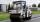Roller has a diameter of 0.96 m and a width 169 cm. How many m2 of road level when he turns 42-times?
2. Axial sectionAxial section of the cylinder has a diagonal 40 cm. The size of the shell and the base surface are in the ratio 3:2. Calculate the volume and surface area of this cylinder.
3. Rotary cylinder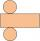The rotating cylinder has a surface area 69.08 cm2. The area of the shell is 62.8 cm 2. What is the diameter of the cylinder?
4. Cylinder - basicsCylinder with base radius r = 45 dm and height h=22 dm. Calculate:
5. Cylinder surface, volumeThe area of the cylinder surface and the cylinder jacket are in the ratio 3: 5. The height of the cylinder is 5 cm shorter than the radius of the base. Calculate surface area and volume of cylinder.
6. Cylinder - A&VThe cylinder has a volume 1287. The base has a radius 10. What is the area of surface of the cylinder?
7. Cylinder diameter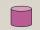The surface of the cylinder is 149 cm2. The cylinder height is 6 cm. What is the diameter of this cylinder?
8. Pipeline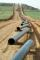How much percent has changed (reduced) area of pipe cross-section, if circular shape changed to square with same perimeter?
9. Triangular prism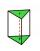The base perpendicular triangular prism is a right triangle whose hypotenuse measures 5 cm and one cathetus 2 cm. Height of the prism is equal to 7/9 of the perimeter of the base. Calculate the surface area of prism.
10. Theorem proveWe want to prove the sentense: If the natural number n is divisible by six, then n is divisible by three. From what assumption we started?
11. Diameters of circlesHow many percent of the area of a larger circle is a smaller circle if the smaller circle has a diameter 120 mm and a larger one has a diameter 300 mm?
12. Quatrefoil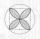Calculate area of the quatrefoil which is inscribed in a square with side 6 cm.
13. AceThe length of segment AB is 24 cm and the point M and N divided it into thirds. Calculate the circumference and area of this shape.
14. Rectangle AntonDifference between length and width of the rectangle is 8. Length is 3-times larger than the width. Calculate the dimensions of the rectangle.
15. Circular lawnAround a circular lawn area is 2 m wide sidewalk. The outer edge of the sidewalk is curb whose width is 2 m. Curbstone and the inner side of the sidewalk together form a concentric circles. Calculate the area of the circular lawn and the result round to 1
16. Reference angleFind the reference angle of each angle:
17. BlocksThere are 9 interactive basic building blocks of an organization. How many two-blocks combinations are there?